# Finding Effective Resistance using Kirchhoff's Rule

## Homework Statement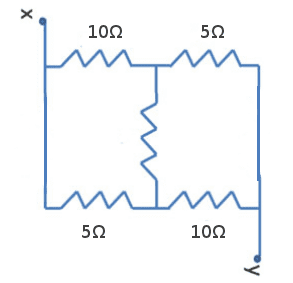Find the effective resistance between the points 'x' and 'y'. When the middle Resistance is 5 ohms.

## Homework Equations

Ohm's Law - V=IR. Kirchhoff's Junction and Loop (Mesh) Rule.

## The Attempt at a Solution

I drew the following diagram: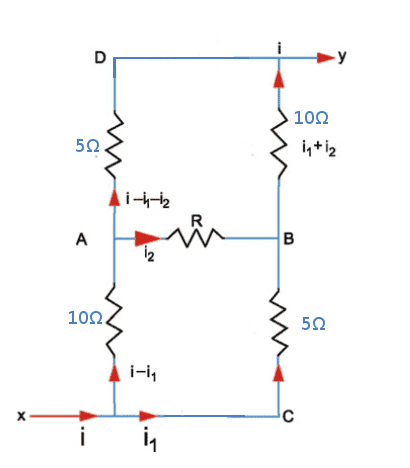I got the correct answer when I took the loops 'ABCA' and 'ADBA' and resolved ##i_1## and ##i_2## (using Kirchhoff's Rules) in terms of ##i## and applied this into an imaginary loop which contains an arbitrary cell of emf ##V## (its terminals connected to 'x' and 'y') and finding ##R_{eq}=V/i##.

But when I tried doing this problem by taking the loops 'xADyx' (which contains an imaginary arbitrary cell) , then the loop 'xAByx' and then 'xCByx' and as above resolve ##i_1## and ##i_2## in terms of ##i## and when I find ##V/i## I get the wrong answer. I tried many times. Is there something I am doing wrong in the second case? Or am I repeatedly making algebraic mistakes?

One more question: My textbook resolves the above diagram using symmetry. In the text they take the current going through both the ##5\Omega## as same and similarly in both the ##10\Omega## resistors as same. How do they do that? How can I find symmetry in a circuit ?

gneill
Mentor

## Homework Statement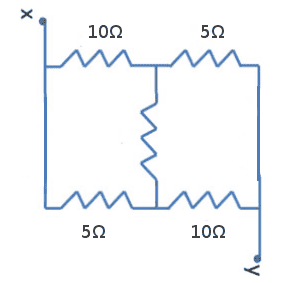Find the effective resistance between the points 'x' and 'y'. When the middle Resistance is 5 ohms.

## Homework Equations

Ohm's Law - V=IR. Kirchhoff's Junction and Loop (Mesh) Rule.

## The Attempt at a Solution

I drew the following diagram: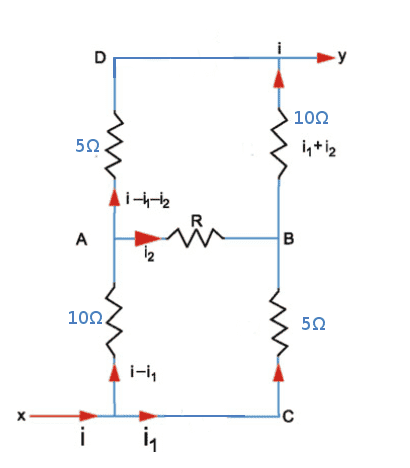I got the correct answer when I took the loops 'ABCA' and 'ADBA' and resolved ##i_1## and ##i_2## (using Kirchhoff's Rules) in terms of ##i## and applied this into an imaginary loop which contains an arbitrary cell of emf ##V## (its terminals connected to 'x' and 'y') and finding ##R_{eq}=V/i##.

But when I tried doing this problem by taking the loops 'xADyx' (which contains an imaginary arbitrary cell) , then the loop 'xAByx' and then 'xCByx' and as above resolve ##i_1## and ##i_2## in terms of ##i## and when I find ##V/i## I get the wrong answer. I tried many times. Is there something I am doing wrong in the second case? Or am I repeatedly making algebraic mistakes?
Do all of your loops contain the 'arbitrary cell'? If not, can you show your loops on a diagram?

You'll have to show your algebra in order for anyone to be able comment on it.
One more question: My textbook resolves the above diagram using symmetry. In the text they take the current going through both the ##5\Omega## as same and similarly in both the ##10\Omega## resistors as same. How do they do that? How can I find symmetry in a circuit ?
It can be a bit of an art, recognizing symmetry in a circuit. Here you could rotate the circuit so that x appears in the top right and y the lower left, and the result would be indistinguishable from the original save for a re-labeling of the nodes. So the same EMF applied in either orientation should yield the same currents for the components. Rotating the circuit and applying the EMF to the result is identical to just swapping the EMF polarity on the original circuit. Current directions may change, but their magnitudes must be the same. These two facts taken together should convince you that the currents in the same-valued resistor pairs must be the equal.

Do all of your loops contain the 'arbitrary cell'? If not, can you show your loops on a diagram?
Yes all the loops I took into consideration contains the arbitrary cell.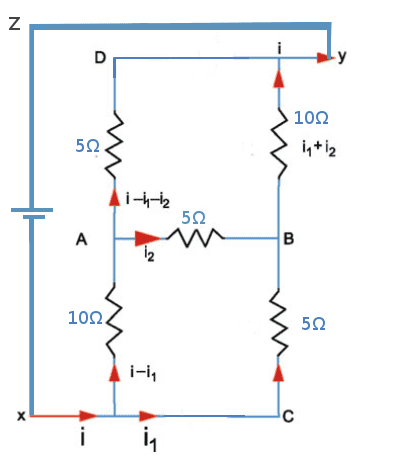I took the loops 'xCByZx', 'xADyZx' and 'xAByZx'.

You'll have to show your algebra in order for anyone to be able comment on it.
The Algebra doesn't need much attention, I would like to know whether I am making any theoretical mistake in the selection of the loops.

Thanks

gneill
Mentor
The Algebra doesn't need much attention, I would like to know whether I am making any theoretical mistake in the selection of the loops.
No, so long as each component in the circuit is passed through at least once by the set of loops you are fine.

I think I covered almost all the components in the circuit considering those three loops. Might be some algebraic error (But I tried it multiple times, never got the correct answer).

gneill
Mentor
I think I covered almost all the components in the circuit considering those three loops. Might be some algebraic error (But I tried it multiple times, never got the correct answer).
"Almost" doesn't work. But I can see that your choice of loops does cover all the components. You should note that your loops do not all have to pass through the voltage source. It is sufficient that all components are covered. So for example: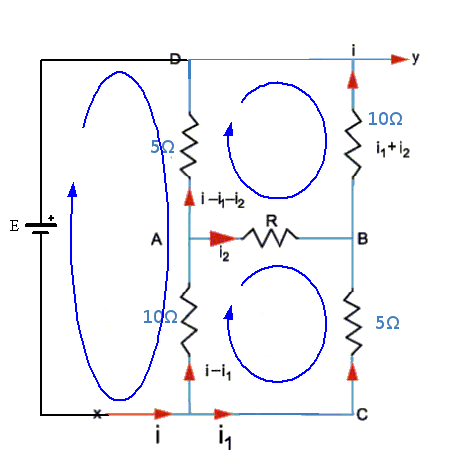would be a perfectly good set of loops to use.

"Almost" doesn't work. But I can see that your choice of loops does cover all the components. You should note that your loops do not all have to pass through the voltage source. It is sufficient that all components are covered. So for example:
View attachment 76786

would be a perfectly good set of loops to use.

I understand that. I got the answer following the above diagram. But I can't find any reason why I am wrong in selecting the loops (all three of them) containing the voltage source. Am just worried whether I am missing out any important point.

ehild
Homework Helper
I understand that. I got the answer following the above diagram. But I can't find any reason why I am wrong in selecting the loops (all three of them) containing the voltage source. Am just worried whether I am missing out any important point.

You can select any independent three loops. Show your equations, please, and then we find your mistake.

NascentOxygen
Staff Emeritus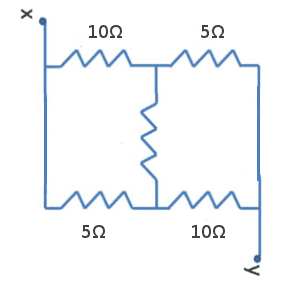One more question: My textbook resolves the above diagram using symmetry. In the text they take the current going through both the ##5\Omega## as same and similarly in both the ##10\Omega## resistors as same. How do they do that? How can I find symmetry in a circuit ?
A little maths can support or dismiss an intuition ......

Imagine a current I1 from x to y through the top two resistors, and a corresponding current I2 through the bottom horizontal pair. Also, let a current IR flow from x to y via the top 10Ω through the unmarked vertical R and the lower 10Ω resistor.

Vxy calculated across the top horizontal resistors must equal the voltage Vxy across the lower horizontal resistors:

10 (I1 + IR) + 5 I1 = 10 (I2 + IR) + 5 I2

The obvious non-zero solution to this is that I1 = I2

So it follows that the current is identical in each 10Ω resistor. Likewise, the 5Ω resistors have equal currents.

To determine the current through the central resistor, calculate the potential difference between the points it connects to, and equate this to R × IR

The figures will reveal that, because of the symmetry, the upper end of R is as many volts less than Vx as the lower end is greater than Vy.
http://thumbnails112.imagebam.com/37333/0363e9373324851.jpg [Broken]

Last edited by a moderator:

## Homework StatementFind the effective resistance between the points 'x' and 'y'. When the middle Resistance is 5 ohms.
If you need just resistance between points x and y, use delta-wye transformation formulae to one of the loops with 3 resistors in the circuit.
It goes much faster this way.

ehild
Homework Helper
Yes all the loops I took into consideration contains the arbitrary cell.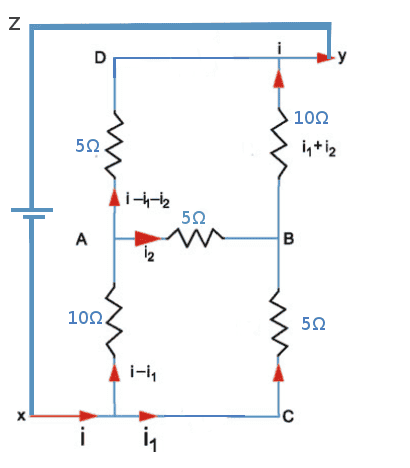I took the loops 'xCByZx', 'xADyZx' and 'xAByZx'.

The Algebra doesn't need much attention, I would like to know whether I am making any theoretical mistake in the selection of the loops.

Thanks
You can choose those loops. If you took the short-line terminal of the source positive, the loop equations are:
E=15I1-10I2
E=15i -15I1-5I2
E=10i+15I2.
What are yours?

epenguin
Homework Helper
Gold Member
I got the correct answer when I

Is there anything noteworthy, striking, simple, about it?

A little maths can support or dismiss an intuition ......

Imagine a current I1 from x to y through the top two resistors, and a corresponding current I2 through the bottom horizontal pair. Also, let a current IR flow from x to y via the top 10Ω through the unmarked vertical R and the lower 10Ω resistor.

Vxy calculated across the top horizontal resistors must equal the voltage Vxy across the lower horizontal resistors:

10 (I1 + IR) + 5 I1 = 10 (I2 + IR) + 5 I2

The obvious non-zero solution to this is that I1 = I2

So it follows that the current is identical in each 10Ω resistor. Likewise, the 5Ω resistors have equal currents.

To determine the current through the central resistor, calculate the potential difference between the points it connects to, and equate this to R × IR

The figures will reveal that, because of the symmetry, the upper end of R is as many volts less than Vx as the lower end is greater than Vy.
http://thumbnails112.imagebam.com/37333/0363e9373324851.jpg [Broken]

NascentOxygen - Thanks! That quite explains why the values of the current are same. But I guess its more challenging to assume that without any mathematics (and that would be handy in competitive examinations.)

If you need just resistance between points x and y, use delta-wye transformation formulae to one of the loops with 3 resistors in the circuit.
It goes much faster this way.

I should try that. Thanks!